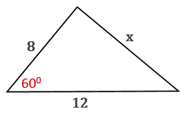Chapter 11.CT, Problem 19CTElementary Geometry For College St...

7th Edition
Alexander + 2 others
ISBN: 9781337614085

Solutions

Chapter
SectionElementary Geometry For College St...

7th Edition
Alexander + 2 others
ISBN: 9781337614085
Textbook Problem

Use the Law of Sines or the Law of Cosines to find length x to the nearest whole number.To determine

To find:

The length x to the nearest whole number by using the Law of Sines or the Law of Cosines.

Explanation

Given:

The diagram as below.

Theorem:

In any acute triangle ABC,

c2=a2+b2-2ab cos γ

b2=a2+c2-2ac cos β

a2=b2+c2-2bc cos α, which is called the Law of Cosines.

Calculation:

From the diagram given,

Let a=x, b=8, c=12 A=650, and α be the angle opposite to a

Still sussing out bartleby?

Check out a sample textbook solution.

See a sample solution

The Solution to Your Study Problems

Bartleby provides explanations to thousands of textbook problems written by our experts, many with advanced degrees!

Get Started

In Exercises 13-20, sketch a set of coordinate axes and plot each point. 16. (3, 4)

Applied Calculus for the Managerial, Life, and Social Sciences: A Brief Approach

Evaluate each expression: 10+42

Elementary Technical Mathematics

Solve the equations in Exercises 112 for x (mentally, if possible). ax+b=c(a0)

Finite Mathematics and Applied Calculus (MindTap Course List)

45 15 63 127

Study Guide for Stewart's Multivariable Calculus, 8th

True or False: f(x) = 10x − x2 is increasing on (4,8).

Study Guide for Stewart's Single Variable Calculus: Early Transcendentals, 8th